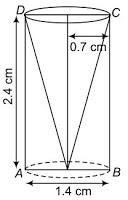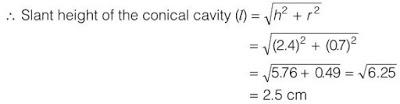Newbie

# From a solid cylinder whose height is 2.4 cm and diameter 1.4 cm, a conical cavity of the same height and same diameter is hollowed out. Find the total surface area of the remaining solid to the nearest cm2. Q.8

• 1

Give me the best method to solve the surface areas and volumes of ncert class 10 , Find the easiest solution of the exercise 13.1 question number 8 . Also find the simplest method of this question . From a solid cylinder whose height is 2.4 cm and diameter 1.4 cm, a conical cavity of the same height and same diameter is hollowed out. Find the total surface area of the remaining solid to the nearest cm2.

Share

1. The diagram for the question is as follows:From the question we know the following:

The diameter of the cylinder = diameter of conical cavity = 1.4 cm

So, the radius of the cylinder = radius of the conical cavity = 1.4/2 = 0.7

Also, the height of the cylinder = height of the conical cavity = 2.4 cmNow, the TSA of remaining solid = surface area of conical cavity + TSA of the cylinder

= πrl+(2πrh+πr2)

= πr(l+2h+r)

= (22/7)× 0.7(2.5+4.8+0.7)

= 2.2×8 = 17.6 cm2

So, the total surface area of the remaining solid is 17.6 cm2

• 1